﻿ 某些具有紧-Gδ性质的空间的函数刻画 Function Characterizations of Some Spaces with Compact Gδ-Property

Pure Mathematics
Vol. 09  No. 01 ( 2019 ), Article ID: 28377 , 5 pages
10.12677/PM.2019.91007

Function Characterizations of Some Spaces with Compact Gδ-Property

Congcong Wu

School of Mathematics & Physics, Anhui University of Technology, Ma’anshan Anhui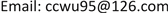Received: Dec. 12th, 2018; accepted: Jan. 1st, 2019; published: Jan. 9th, 2019ABSTRACT

Real-valued functions are useful tools for the characterizations of some topological spaces. Many classes of spaces can be characterized with real-valued functions that satisfy certain conditions, such as stratifiable spaces, k-semi-stratifiable spaces, etc. In this paper, we present some characterizations of spaces with compact Gδ-property in terms of real-valued functions, such as γ-spaces, c-stratifiable spaces, kc-stratifiable spaces, etc. The results obtained generalize some corresponding results for stratifiable spaces, k-semi-stratifiable spaces in the literature.

Keywords:γ-Spaces, C-Stratifiable Spaces, Kc-Stratifiable Spaces, Semi-Continuous Functions1. 前言

2. 主要结果

(1) $\underset{n\to \infty }{\mathrm{lim}}{\delta }_{nK}={\chi }_{K}$

(2) 若 ${K}_{1},{K}_{2}\in C\left(X\right)$${K}_{1}\subset {K}_{2}$，则对每一 $n\in N$${\delta }_{n{K}_{1}}\le {\delta }_{n{K}_{2}}$

(3) 对每一紧集 $K\in X$$F\in {\tau }^{c}$$K\cap F=\Phi$，存在 $m\in N$ 使得对任意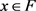${\delta }_{mK}\left(x\right)=0$

(1) 设 $K\in C\left(X\right)$，对每一 $x\in X$，若 $x\in K$，则对每一 $n\in N$$x\in g\left(n,K\right)$，于是 $\underset{n\to \infty }{\mathrm{lim}}{\delta }_{nk}\left(x\right)=1={\chi }_{k}\left(x\right)$；若 $x\notin K$，则存在 $m\in N$ 使得 $\left\{x\right\}\cap g\left(m,K\right)=\Phi$，于是当 $n\ge m$ 时，有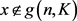，故 $\underset{n\to \infty }{\mathrm{lim}}{\delta }_{nK}\left(x\right)=0={\chi }_{K}\left(x\right)$

(2) 设 ${K}_{1},{K}_{2}\in C\left(X\right)$${K}_{1}\subset {K}_{2}$，则对每一 $n\in N$，有 $g\left(n,{K}_{1}\right)\subset g\left(n,{K}_{2}\right)$，由此可得 ${\delta }_{n{K}_{1}}\le {\delta }_{n{K}_{2}}$

(3) 设 $K\in C\left(X\right)$$F\in {\tau }^{c}$$K\cap F=\Phi$，由引理2.1，存在 $m\in N$ 使得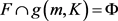，故对任意 $x\in F$，有 $x\notin g\left(m,K\right)$，则 ${\delta }_{mk}\left(x\right)=0$

(1) 对每一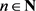$K\in C\left(X\right)$，若 $x\in K$，则 ${\delta }_{nK}\left(x\right)=1$

(2) 若 ${K}_{1},{K}_{2}\in C\left(X\right)$${K}_{1}\subset {K}_{2}$，则对每一 $n\in N$，有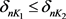(3) 若 $K\in C\left(X\right)$$F\in {\tau }^{c}$$K\cap F=\Phi$，则 $〈{\delta }_{nK}〉$$F$ 上一致收敛于0。

(3)设 $K\in C\left(X\right)$$F\in {\tau }^{c}$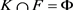，由引理2.1，存在 $m\in N$ 使得 $F\cap g\left(m,K\right)=\Phi$。对 $\forall \epsilon >0$，当 $n\ge m$ 时，对任意 $x\in F$$x\notin g\left(n,K\right)$，故 $|{\delta }_{nk}\left(x\right)-0|<\epsilon$，这说明 $〈{\delta }_{nK}〉$$F$ 上一致收敛于0。

(1) $\underset{n\to \infty }{\mathrm{lim}}{\delta }_{nK}={\chi }_{K}$

(2) 若 ${K}_{1},{K}_{2}\in C\left(X\right)$ 且若 ${K}_{1}\subset {K}_{2}$，则对每一 $n\in N$，有 ${\delta }_{n{K}_{1}}\le {\delta }_{n{K}_{2}}$

(3) 若 $K\in C\left(X\right)$$F\in {\tau }^{c}$$K\cap F=\Phi$，则存在开集 $V\supset F$$m\in N$ 使得对任意 $x\in V$
${\delta }_{mK}\left(x\right)=0$

(1) $\underset{n\to \infty }{\mathrm{lim}}{\delta }_{nK}=\underset{n\to \infty }{\mathrm{lim}}{\zeta }_{nK}={\chi }_{K}$

(2) 若 ${K}_{1},{K}_{2}\in C\left(x\right)$${K}_{1}\subset {K}_{2}$，则对每一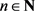${\delta }_{n{K}_{1}}\le {\delta }_{n{K}_{2}}$

(3) 对每一 $K\in C\left(X\right)$$n\in N$${\delta }_{nK}\le {\zeta }_{nK}$

(1) 设 $x\in X$，若 ${\chi }_{K}\left(x\right)=1$，则 $x\in K$，则对每一 $n\in N$，有 $x\in g\left(n,K\right)\subset \overline{g\left(n,K\right)}$，则 $\underset{n\to \infty }{\mathrm{lim}}{\delta }_{nK}\left(x\right)=\underset{n\to \infty }{\mathrm{lim}}{\zeta }_{nK}\left(x\right)=1={\chi }_{K}\left(x\right)$；若 ${\chi }_{K}\left(x\right)=0$，则 $x\notin K$，故存在 $m\in n$ 使得对任意 $n\ge m$，有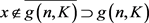，于是 $\underset{n\to \infty }{\mathrm{lim}}{\delta }_{nK}\left(x\right)=\underset{n\to \infty }{\mathrm{lim}}{\zeta }_{nK}\left(x\right)=0={\chi }_{K}\left(x\right)$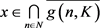，则对每一 $n\in N$$x\in \overline{g\left(n,K\right)}\subset F\left(n,K\right)$，则 ${\chi }_{K}\left(x\right)=\underset{n\to \infty }{\mathrm{lim}}{\zeta }_{nK}\left(x\right)\ge 1/2$，故
$x\in K$，这说明 $\underset{n\in N}{\cap }\overline{g\left(n,K\right)}\subset K$，于是 $K=\underset{n\in N}{\cap }\overline{g\left(n,K\right)}$。故 $X$ 为c-层空间。

(1)(2) 若 ${K}_{1},{K}_{2}\in C\left(x\right)$${K}_{1}\subset {K}_{2}$，则对每一 $n\in N$，有 ${\delta }_{n{K}_{1}}\le {\delta }_{n{K}_{2}}$

(3)若 $K,H\in C\left(x\right)$$K\cap H=\Phi$，则存在 $m\in N$ 使得对任意 $x\in H$${\delta }_{mK}\left(x\right)=0$

(1) 对每一 $n\in N$$K\in C\left(x\right)$，若 $x\in K$，则 ${\delta }_{nK}\left(x\right)=1$

(2) 若 ${K}_{1},{K}_{2}\in C\left(x\right)$${K}_{1}\subset {K}_{2}$，则对每一 $n\in N$，有 ${\delta }_{n{K}_{1}}\le {\delta }_{n{K}_{2}}$

(3) 若 $K,H\in C\left(x\right)$，且 $K\cap H=\Phi$，则 $〈{\delta }_{nK}〉$$H$ 上一致收敛于0。

(1) $\underset{n\to \infty }{\mathrm{lim}}{\delta }_{nK}={\chi }_{K}$

(2) 若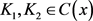${K}_{1}\subset {K}_{2}$，则对每一 $n\in N$，有 ${\delta }_{n{K}_{1}}\le {\delta }_{n{K}_{2}}$

Function Characterizations of Some Spaces with Compact Gδ-Property[J]. 理论数学, 2019, 09(01): 49-53. https://doi.org/10.12677/PM.2019.91007

1. 1. Engelking, R. (1977) General Topology. Polish Scientific Publishers, Warszawa.

2. 2. Tong, H. (1952) Some Characterizations of Normal and Perfectly Normal Spaces. Duke Mathematical Journal, 19, 289-292.
https://doi.org/10.1215/S0012-7094-52-01928-5

3. 3. 张国芳, 杨二光. k-半层空间的函数刻画[J]. 山东大学学报(理学报), 2006, 26(2): 213-218.

4. 4. Hodel, R.E. (1972) Hodel, Spaces Defined by Sequence of Open Covers Which Guarantee that Certain Sequences Have Cluster Points. Duke Mathematical Journal, 39, 253-263.
https://doi.org/10.1215/S0012-7094-72-03930-0

5. 5. Martin, H.W. (1973) Metrizability of M-Spaces. Canadian Journal of Mathematics, 25, 840-841.
https://doi.org/10.4153/CJM-1973-086-0

6. 6. Martin II, H.W. (1973) Metrization and Submetrization of Topological Spaces. Ph D Thesis, University of Pittsburgh, Pittsburgh, PA.

7. 7. Lindgren, W.F. and Fletcher, P. (1974) Locally Quasi-Uniform Spaces with Countable Bases. Duke Mathematical Journal, 41, 231-240.
https://doi.org/10.1215/S0012-7094-74-04125-8